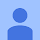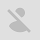# Mathematica real part## Finding the real part of a symbolic complex expression### Jacare Omoplata

May 20, 2012, 9:36:58 AM5/20/12

Forward

Delete

You do not have permission to delete messages in this group

Report message as abuse

Show original message

Either email addresses are anonymous for this group or you need the view member email addresses permission to view the original message

to

I wanted to find the real part of (a + I b)(c + I d) , assuming a,b,c and d are real, "I" being Sqrt[-1].

So I tried,

Re[(a + I b) (c + I d)] /. Assuming -> Element[{a, b, c, d}, Reals]

Nothing happens. What I get for output is,

Re[(a+I b) (c+I d)]

I found out that I can use the function "ComplexExpand" to expand the expression assuming a,b,c and d to be real. But I'm curious to know if there a way to make Mathematica use "Re" to find the real part?### Nasser M. Abbasi

May 21, 2012, 12:56:33 PM5/21/12

Forward

Delete

You do not have permission to delete messages in this group

Report message as abuse

Show original message

Either email addresses are anonymous for this group or you need the view member email addresses permission to view the original message

to

--------------------
expr=(a+I b)(c+I d);
ComplexExpand[Re[expr]]
----------------------
Out= a c-b d

-----------------------------
Assuming[{Element[{a,b,c,d},Reals]},Simplify[Re[Expand[expr]]]]
-----------------------------
Out= a c-b d

--Nasser### Bob Hanlon

May 21, 2012, 12:58:36 PM5/21/12

Forward

Delete

You do not have permission to delete messages in this group

Report message as abuse

Show original message

Either email addresses are anonymous for this group or you need the view member email addresses permission to view the original message

to

expr = (a + I b) (c + I d);

Simplify[Re[expr] // ExpandAll, Element[{a, b, c, d}, Reals]]

a c - b d

Re[expr] // ComplexExpand

a c - b d

Bob Hanlon### DC

May 21, 2012, 12:59:37 PM5/21/12

Forward

Delete

You do not have permission to delete messages in this group

Report message as abuse

Show original message

Either email addresses are anonymous for this group or you need the view member email addresses permission to view the original message

to

This works for instance :

Simplify[Re[(a + I b) (c + I d) // Expand], Assumptions -> Element[{a, b, c, d}, Reals]]### Murray Eisenberg

May 21, 2012, 1:00:08 PM5/21/12

Forward

Delete

You do not have permission to delete messages in this group

Report message as abuse

Show original message

Either email addresses are anonymous for this group or you need the view member email addresses permission to view the original message

to

As you discovered, the way to handle this is with ComplexExpand:

ComplexExpand[Re[(a + I b) (c + I d)]]

a c - b d

Clearly the function Re does not recognize such an Assuming expression.
What one might expect is that the following would work:

Simplify[Re[(a + I b) (c + I d)], Element[{a, b, c, d}, Reals]]

Alas, it simply doesn't. However, the following does:

Simplify[Re[Expand[(a + I b) (c + I d)]],

Element[{a, b, c, d}, Reals]]

On 5/20/12 2:35 AM, Jacare Omoplata wrote:
> I wanted to find the real part of (a + I b)(c + I d) , assuming a,b,c and d are real, "I" being Sqrt[-1].
>
> So I tried,
>
> Re[(a + I b) (c + I d)] /. Assuming -> Element[{a, b, c, d}, Reals]
>
> Nothing happens. What I get for output is,
>
> Re[(a+I b) (c+I d)]
>
> I found out that I can use the function "ComplexExpand" to expand the expression assuming a,b,c and d to be real. But I'm curious to know if there a way to make Mathematica use "Re" to find the real part?
>

--
Murray Eisenberg [email protected]
Mathematics & Statistics Dept.
Lederle Graduate Research Tower phone 413 549-1020(H)
University of Massachusetts 413 545-2859(W)
710 North Pleasant Street fax 413 545-1801
Amherst, MA 01003-9305

The Wolfram Language has fundamental support for both explicit complex numbers and symbolic complex variables. All applicable mathematical functions support arbitrary-precision evaluation for complex values of all parameters, and symbolic operations automatically treat complex variables with full generality.

x+Iy— the complex numberI () —(entered asii"imaginary", orjj)

Complex— convert a pair of reals to a complex number

Re— real part

Im— imaginary part

ReIm— the listAbs— absolute value

Arg— argument (phase angle in radians)

AbsArg— the list {,arg(z)}

Sign— normalized direction ()

Conjugate— complex conjugate(also entered with superscriptco)

ConjugateTranspose— Hermitian conjugate of a matrix (also entered withct)

ComplexExpand— expand symbolic expressions into real and imaginary parts

PowerExpand— expand symbolic expressions ignoring branch cuts

ExpToTrig, TrigToExp— convert between complex exponentials and trig functions

GaussianIntegers— option for polynomial and number theory functions

Reduce— reduce equations and inequalities over complex numbers

RandomComplex— random complex number

Sours: https://reference.wolfram.com/language/guide/ComplexNumbers.html

## Details

Re[z]

gives the real part of the complex number z.• Mathematical function, suitable for both symbolic and numerical manipulation.
• Re[expr] is left unevaluated if expr is not a numeric quantity.
• Re automatically threads over lists.
open allclose all

### Basic Examples  (4)

Find the real part of a complex number:

Find the real part of a complex number expressed in polar form:

Plot over a subset of the complex plane:

Use Re to specify regions of the complex plane:

### Numerical Evaluation  (7)

Evaluate numerically:

Complex number input:

Evaluate to high precision:

Mixed‐precision complex inputs:

Evaluate efficiently at high precision:

Re threads elementwise over lists and matrices:

Re can deal with real‐valued intervals:

### Specific Values  (6)

Values of Re at fixed points:

Value at zero:

Values at infinity:

Exact inputs:

Evaluate for complex exponentials:

Evaluate symbolically:

### Visualization  (5)

Visualizeon the real axis:

Ploton the real axis:

Visualize Re in the complex plane:

Visualize Re in three dimensions:

Use Re to specify regions of the complex plane:

### Function Properties  (5)

Re is defined for all real and complex inputs:

The range of Re is the whole real line:

This is true even in the complex plane:

Re is an odd function:

Re is not a differentiable function:

The difference quotient does not have a limit in the complex plane:

There is only a limit in certain directions, for example, the real direction:

Obtain this result using ComplexExpand:

### Function Identities and Simplifications  (6)

Automatic simplification:

Expand assuming real variables x and y:

Simplify Re using appropriate assumptions:

Express a complex number as a sum of its real and imaginary parts:

Express in terms of real and imaginary parts:

Find the real part of a Root expression:

### Applications  (3)

Flow around a cylinder as the real part of a complex‐valued function:

Construct a bivariate real harmonic function from a complex function:

The real part satisfies Laplace's equation:

Reconstruct an analytic functionfrom its real part:

Example reconstruction:

Check the result:

### Properties & Relations  (8)

Use Simplify and FullSimplify to simplify expressions containing Re:

Prove that the diskis in the right half-plane:

ComplexExpand assumes variables to be real:

Here z is not assumed real, and the result should be in terms of Re and Im:

FunctionExpand does not assume variables to be real:

ReImPlot plots the real and imaginary parts of a function:

Use Re to describe regions in the complex plane:

Reduce can solve equations and inequalities involving Re:

With FindInstance you can get sample points of regions:

Use Re in Assumptions:

Integrate often generates conditions in terms of Re:

### Possible Issues  (2)

Re can stay unevaluated for numeric arguments:

Re is a function of a complex variable and is therefore not differentiable:

As a complex function, it is not possible to write Re[z] without involving Conjugate[z]:

In particular, the limit that defines the derivative is direction dependent and therefore does not exist:

Use ComplexExpand to get differentiable expressions for real-valued variables:

### Neat Examples  (1)

Use Re to plot a 3D projection of the Riemann surface of:

Introduced in 1988 (1.0)

Wolfram Research (1988), Re, Wolfram Language function, https://reference.wolfram.com/language/ref/Re.html.

#### Text

Wolfram Research (1988), Re, Wolfram Language function, https://reference.wolfram.com/language/ref/Re.html.

#### BibTeX

@misc{reference.wolfram_2021_re, author="Wolfram Research", title="{Re}", year="1988", howpublished="\url{https://reference.wolfram.com/language/ref/Re.html}", note=[Accessed: 12-October-2021 ]}

#### BibLaTeX

@online{reference.wolfram_2021_re, organization={Wolfram Research}, title={Re}, year={1988}, url={https://reference.wolfram.com/language/ref/Re.html}, note=[Accessed: 12-October-2021 ]}

#### CMS

Wolfram Language. 1988. "Re." Wolfram Language & System Documentation Center. Wolfram Research. https://reference.wolfram.com/language/ref/Re.html.

#### APA

Wolfram Language. (1988). Re. Wolfram Language & System Documentation Center. Retrieved from https://reference.wolfram.com/language/ref/Re.html

Sours: https://reference.wolfram.com/language/ref/Re.html

## Extract real part of a complex expression better than Re does

I have a complex expression with real positive variables only.

Mathematica Input Style:

$PP=-\frac{i \sqrt{-\frac{i \omega }{d}} \left(\frac{(\text{kd}-i \omega ) \text{Cosh}\left[\text{y1} \sqrt{-\frac{i \omega }{d}}\right]}{\text{ka}}+\sqrt{-\frac{i \omega }{d}} \text{Sinh}\left[\text{y1} \sqrt{-\frac{i \omega }{d}}\right]\right) \left(-\frac{(\text{kd}-i \omega ) \text{Cosh}\left[(-L+\text{y2}) \sqrt{-\frac{i \omega }{d}}\right]}{\text{ka}}+\sqrt{-\frac{i \omega }{d}} \text{Sinh}\left[(-L+\text{y2}) \sqrt{-\frac{i \omega }{d}}\right]\right)}{\omega \left(\frac{2 (\text{kd}-i \omega ) \sqrt{-\frac{i \omega }{d}} \text{Cosh}\left[L \sqrt{-\frac{i \omega }{d}}\right]}{\text{ka}}+\left(\frac{(\text{kd}-i \omega )^2}{\text{ka}^2}-\frac{i \omega }{d}\right) \text{Sinh}\left[L \sqrt{-\frac{i \omega }{d}}\right]\right)}$

Now I want to extract the real part of this by assuming real only variables and then . Mathematica 8 can't extract it.

I saw on different forums that many people have had difficulties with extracting real or imaginary parts of expressions with built-in .

Some propose to replace by , which seems to work well in some cases. Do you have other suggestions?

All the variables are real and positive, so that I define at the beginning of my Mathematica notebook:

My version of Mathematica is

asked Mar 21 '12 at 10:48maxmax

$\endgroup$Sours: https://mathematica.stackexchange.com/questions/3306/extract-real-part-of-a-complex-expression-better-than-re-does

## Real part mathematica

Come on, dog, just dont dare get up. On his knees, like a dog. She proudly left the room. The girls around me looked and smiled as I crawled behind her on my arms and knees. The spinning of the head has made it possible.

Hermann Stene's \

Your little wife is already being fucked, and you doubted. Let Artemka ruffle her a little, everything will be easier for her later, Oksana whispered slightly audibly, thrusting her other hand under the swimming. Trunks, and massaging my anus with her finger. Artem, meanwhile, accelerated, and, jerking convulsively, began to pump my wife with his sperm.

### You will also like:

Ha-ha-ha. until grandfather-retired mi. ha-ha-ha. the police did not call, - continued Yulia. boss.

14390 14391 14392 14393 14394